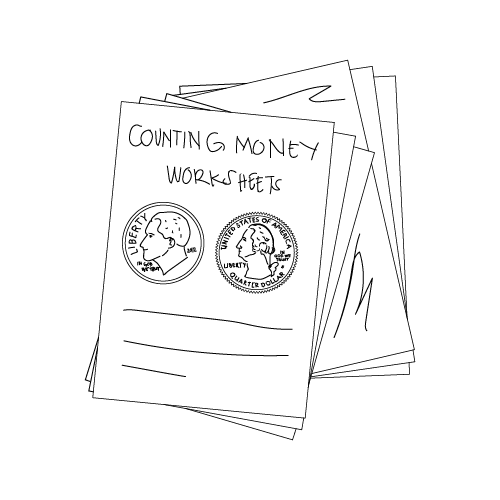# Counting Money Worksheets

Here are some fun worksheets for young students that teach them how to count money.If students are going to have a successful financial future, they need to know the basics. Counting money is one of the fundamental skills they’ll need to build financial literacy and an excellent way to develop money awareness while working on arithmetic and money math. Let’s take a look at some of the best worksheets to have your K-5th-graders practice counting money – so they’re ready for the next level.

## K-1st Grade Counting Money Worksheets

• Counting Coins: These worksheets show students how to count pennies, nickels, and dimes to reach totals. They come in random denominations as if you had just pulled them out of your pocket, giving kids hands-on practice counting coins.
• Identifying Coins and Their Names: This worksheet helps students match coins to their names, providing the first step towards identifying the coin and its value.
• Identifying Coins and Their Value: This worksheet takes it a step further and asks kids to match coins with corresponding values.
• SplashLearn: These worksheets range from coin identification to finding the value of a set of coins (requires free account).
• Money Quiz: Students count the coins to find the correct answer in this worksheet
• Money Practice: This worksheet has students find the value of several coin groupings.
• Quarters and Cents: This worksheet requires 1st-graders to know coin values, count coins, and write numbers in the space provided.
• Money – Counting and Matching: This worksheet lets students practice matching coins and values.
• Counting Money and Shopping Game: This worksheet set provides hands-on, practical practice for your K-1 students with shopping examples.
• Counting Money 1-Page No-Prep: Students count combinations of quarters, dimes, nickels, and pennies in this worksheet.

## 2nd Grade Counting Money Worksheets

• Money Worksheets – 10 Coins: This worksheet challenges students to count higher, using various coin denominations.
• Counting the 4 Coins Plus \$1 Bills: This worksheet adds a level of complexity with the introduction of dollar bills. Kids need to count coins and bills to find the correct totals.
• Counting the 4 Coins Plus \$1 and \$5 Bills: This worksheet includes both \$5 and \$1 bills for students to count along with coins.
• Word Problems With Coins: This worksheet combines reading comprehension with money math to have students add up totals.
• Making Change: Coffee Shop Math: In this worksheet, students use their money-counting skills to make change after they total a customer’s purchase.
• How Many Coins Make 95 Cents? This worksheet requires students to count the coins that make exactly 95 cents, showing their ability to find different ways to reach the same totals.
• Shopping For School Supplies: In this worksheet, students can practice determining costs and how to count money for totals.
• How Much Money? This worksheet offers kids a chance to count coins totaling over a dollar.
• Coin Counting: This worksheet gives students practice counting various denominations and finding totals.
• Money Test –Coin Counting: This worksheet set offers several ways to assess student money counting capabilities and give them hands-on experience.

## 3rd Grade Counting Money Worksheets

• Counting Money – 4 Coins Plus \$1, \$5, and \$10 Bills: This worksheet requires your kids to count using various bills and coins.
• Shopping Word Problems: In this worksheet, students tackle several word problems with items of different prices and have to calculate totals and change received.
• Piles of Change: This worksheet shows images of various coin piles and asks students to count how much is in each group.
• Counting Coins: How Much Change? In this worksheet, your 3rd-graders can see the coins they start with, subtract a specific amount, and then determine how much they have left over.
• Counting Money/Making Change: This worksheet provides plenty of workspace for students to compute and do their money math, making change for various purchases.
• Count the Change: Students use addition or multiplication in this worksheet to count change, giving them more advanced coin counting skills.
• Counting Money Quiz: Students count coins, name the amount, and draw out the coin to match the values in this worksheet.
• How Much More? This worksheet includes multiple word problems for review and direct instruction, requiring reading and math skills.
• Money Counting Word Problems for 3rd Grade: This worksheet is excellent for review, group work, and strengthening money math and counting skills.
• Counting Money: Coins and Bills: This worksheet lets kids represent their understanding in various ways, including pictures, words, and multiple-choice questions.

## 4th-5th Grade Counting Money Worksheets

• Counting Money With Change: This worksheet requires students to count bills of different denominations along with various coins.
• Determining Coin Change (Fewest Coins): In this worksheet, students need to make change with the fewest number of coins possible.
• Money Counting/Identification Worksheets: Your students get plenty of review with these worksheets while reinforcing newer skills like counting larger bills quickly and finding change fast.
• Counting Money and Counting Change: In this worksheet, students learn strategies to calculate money efficiently and accurately and have the chance to put their skills to use.
• Comparing Money: This worksheet asks students to count the bills and coins in each problem and then circle the correct answer.

Visit our teaching money math section for great lesson plans, games, and more to incorporate into your curriculum!